# Create a Sankey Diagram in Python

## Visualize Your Process Data with Sankey Diagram

Sankey diagrams are a great way to visualize processes or flows. Each entity or process stage is represented by nodes. Flows between nodes are expressed in arcs, and the numerical size of the flow determines the size of this arc.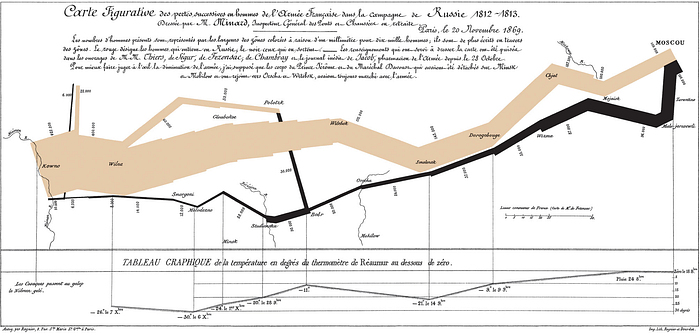Minard's classic diagram of Napoleon's invasion of Russia, using the feature now named after Sankey

In this diagram type, the data to be fed to the chart object must consist of links and nodes. Links define arc connections. These links have directions (from -> to) and values that determines the size of the arc. Nodes are the labels.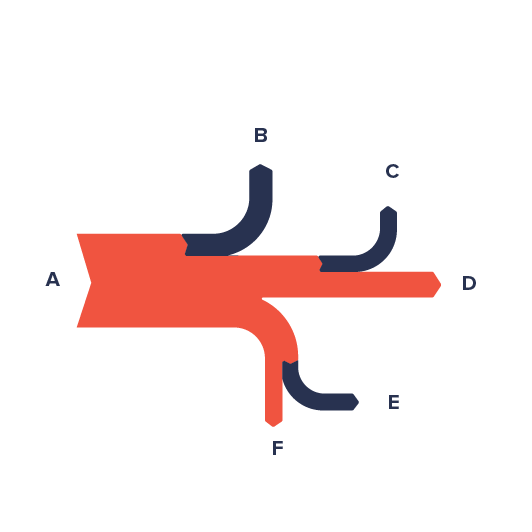Anatomy. Source: https://datavizproject.com/data-type/sankey-diagram/

Let's use a dummy process dataset as an example. A car manufacturer company buys parts from 13 different manufacturers and assembles them in 2 assembly lines to obtain the final product. These vehicles are distributed to the world using 5 different logistics lines. However, according to the reports from car dealers, some vehicles were found to be problematic.

``````import pandas as pd

``````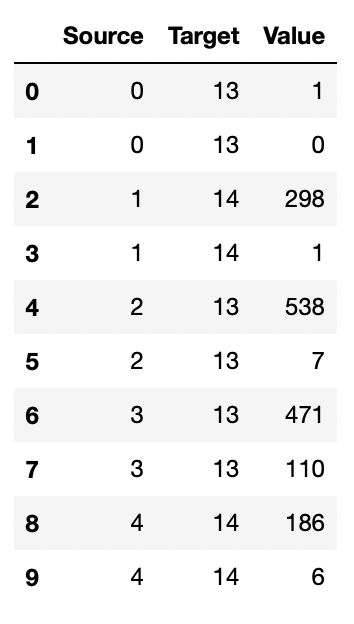Dataset

``````data.tail(10)
``````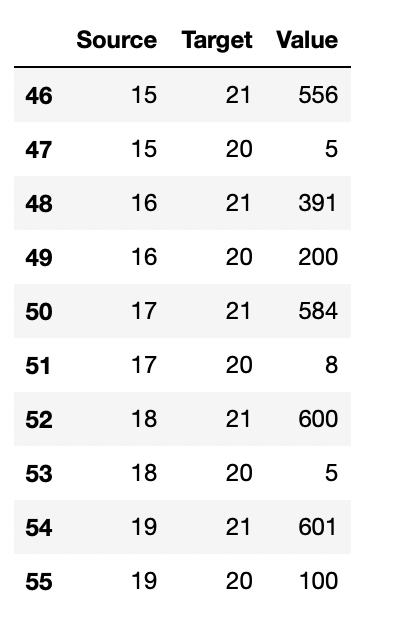``````print(df_labels)
``````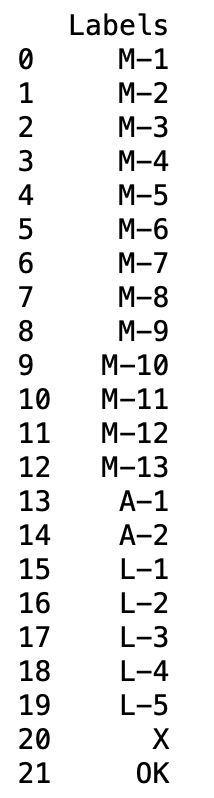Labels

By collecting the necessary data, we can visualize the flow with the Sankey diagram.

``````source = data["Source"].values.tolist()
target = data["Target"].values.tolist()
value = data["Value"].values.tolist()
labels = df_labels["Labels"].values.tolist()
``````

Now let's create the diagram. I will use the Plotly library.

``````import plotly.graph_objs as go

color=["turquoise","tomato"] * len(source))

#create nodes

#create a sankey object

#build a figure
fig = go.Figure(chart)
fig.show()
``````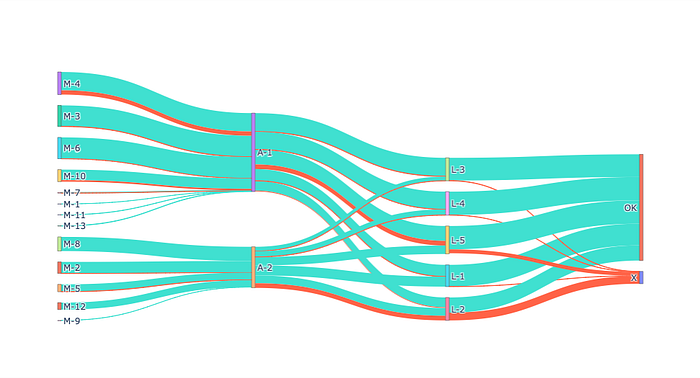Sankey Diagram. Image by the author.

By using the Sankey diagram, we have visually expressed the problems in the process flow. It looks like there is a problem with the manufacturer coded M-4 and the assembly line coded A-2.

### References

Sankey Diagram | Data Viz Project

Sankey

Sankey diagram - Wikipedia